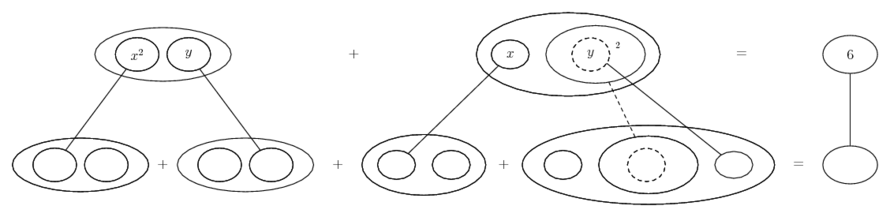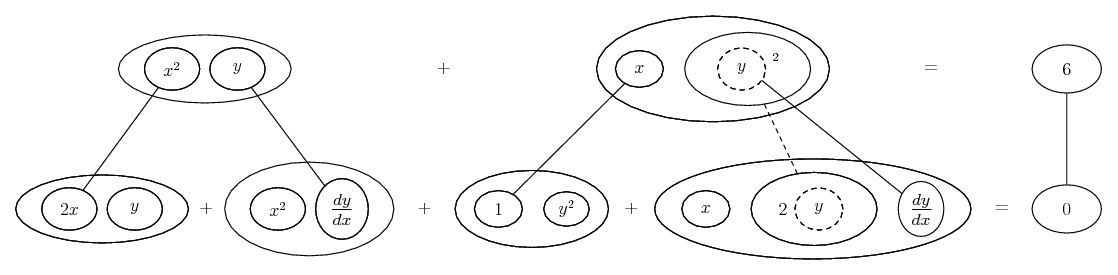# Thread: Implicit differentiation

1. ## Implicit differentiation

Q: Use implicit differentiation to find an equation of the line tangent to the curve yx^2 + xy^2 = 6 at the point (1, 2).

Here's my answer... it is right?

2x(d/dx) + 2y(d/dx) = 6(d/dx) = 0 --> (d/dx)(2x + 2y) = 0 --> dy/dx = 0/(2x + 2y) = 0 = m

y = mx + b = 0x + b = b = 2 --> y =2

2. Just in case a picture helps...Spoiler:Then solve the bottom row for dy/dx and plug in the x and y values for the given point to evaluate m at the point.... is the product rule, and...... the chain rule. Straight continuous lines differentiate downwards (integrate up) with respect to x, and the straight dashed line similarly but with respect to the dashed balloon expression (the inner function of the composite which is subject to the chain rule).

_________________________________________
Don't integrate - balloontegrate!

Balloon Calculus; standard integrals, derivatives and methods

Balloon Calculus Drawing with LaTeX and Asymptote!

3.Originally Posted by satxQ: Use implicit differentiation to find an equation of the line tangent to the curve yx^2 + xy^2 = 6 at the point (1, 2).

Here's my answer... it is right?

2x(d/dx) + 2y(d/dx) = 6(d/dx) = 0 --> (d/dx)(2x + 2y) = 0 --> dy/dx = 0/(2x + 2y) = 0 = m

y = mx + b = 0x + b = b = 2 --> y =2
note that you need the product rule here! y is treated as a function of x. you are to differentiate as usual, but when you differentiate a y-term, you must attach dy/dx to it (this follows from the chain rule). Hence,

$\displaystyle yx^2 + xy^2 = 6$

$\displaystyle \Rightarrow 2xy + x^2 \cdot \frac {dy}{dx} + y^2 + 2xy \cdot \frac {dy}{dx} = 0$

Now solve for $\displaystyle \frac {dy}{dx}$ and plug in $\displaystyle x = 1,~y = 2$. This will give you the $\displaystyle m$ (that is, the slope) for your line

Then use $\displaystyle y - y_1 = m(x - x_1)$ with $\displaystyle (x_1,y_1)= (1,2)$ and the $\displaystyle m$ found above. Solve for $\displaystyle y$ and that's the equation of the tangent line

#### Search Tags

differentiation, implicit